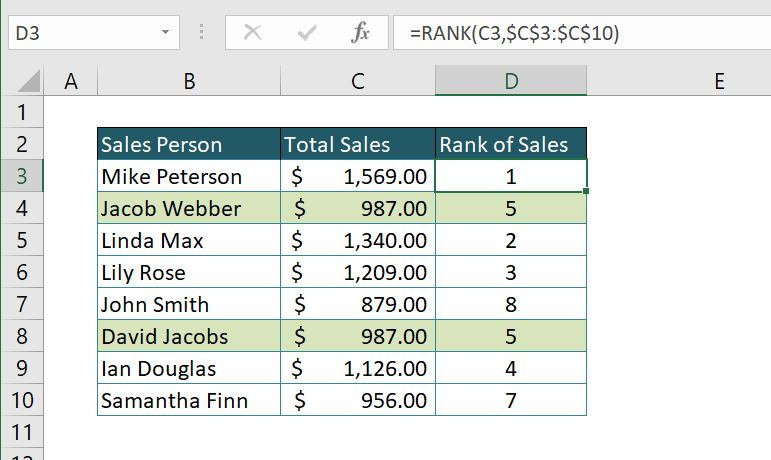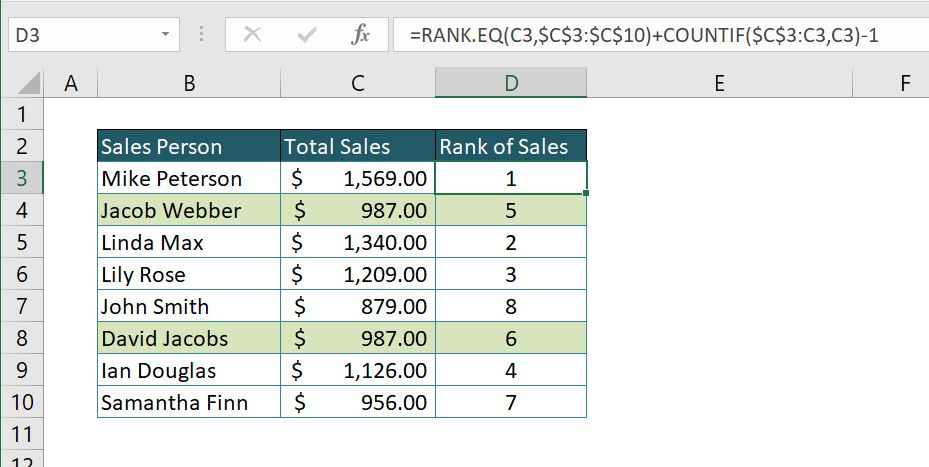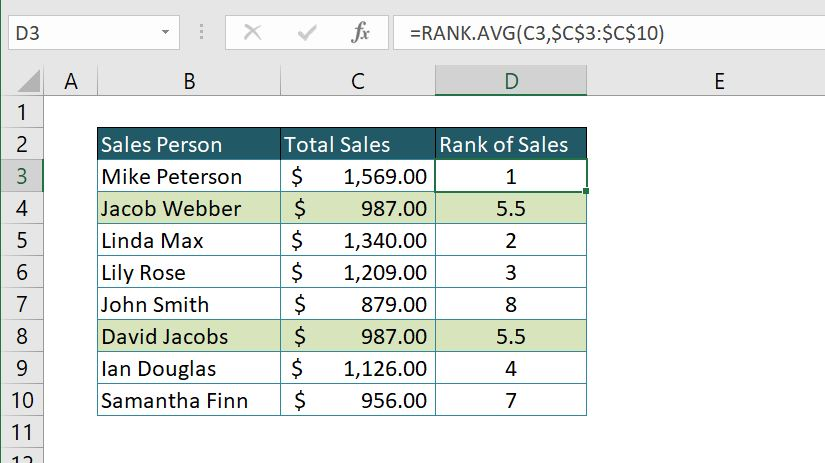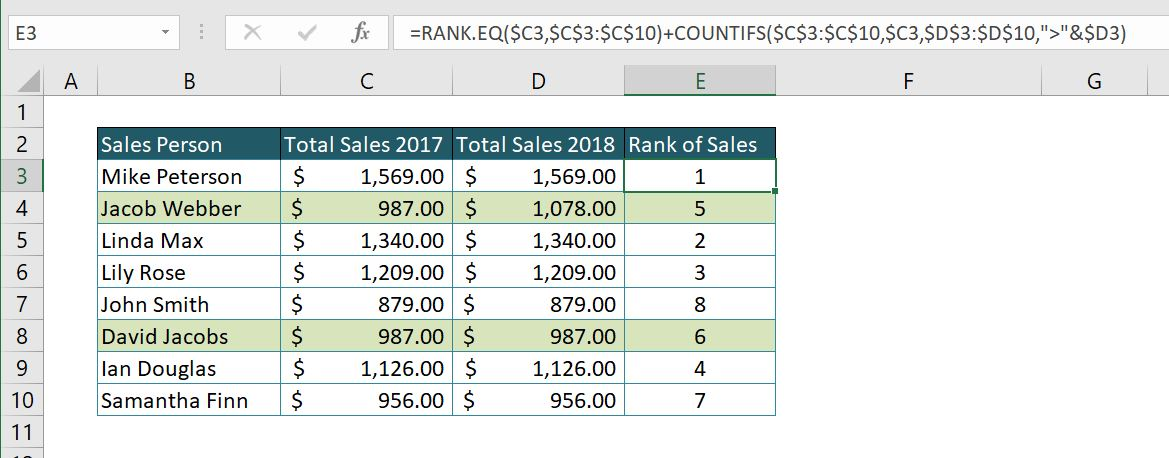Get instant live expert help with Excel or Google Sheets“My Excelchat expert helped me in less than 20 minutes, saving me what would have been 5 hours of work!”

#### Post your problem and you’ll get expert help in seconds.

Your message must be at least 40 characters
Our professional experts are available now. Your privacy is guaranteed.

# How to Perform Excel RANK From Lowest to Highest FunctionRead time: 40 minutes

If you want to find the position of a cell in the defined list of cells, you should use Excel RANK lowest to highest function. This function allows you to determine the position of a certain number in number arrays. In this tutorial, you will see how to rank chosen cell, how to get unique or average rank and how to rank number based on multiple conditions. All of these are useful functions in daily Excel usage.

## Using the RANK Function in Excel

There are several ways to use rank figures in Excel and combine other function with the RANK function.

1. Basic RANK Function
2. EQ Function
3. AVG Function
4. Rank based on multiple conditions

### Basic RANK Function

If you need to rank the position of the certain cell in the selected array of number cells, you should use the RANK function. It returns the position of the chosen number in the list of cells. The syntax of the function looks like:

=NUMBER(Number, Ref, [order])

The number is the cell for which we want to find the position in a list. Ref is the list of numbers (cells) in which we are determining the position of the chosen cell. The order is a non-mandatory parameter of the function and allows us to decide if we want to get a position from ascending or descending sorted list. If we put “0”, that means descending, and “1” means ascending. If this parameter is omitted, the default value is “0” – descending order. Let’s now look at one example of basic usage of RANK function:

`=RANK(C3,\$C\$3:\$C\$10)`

In this example, we want to find the position of Salesperson based on his/her sales volume. Our number will be cell C3 and Ref (list of numbers) C3:C10 (Be sure to put “\$” or press F4 button in this range, because the function will be copied to all rows). In this case, we omitted the Order parameter, which means that we want a descending order. The result is rank number one for the first cell (C3) – which is the greatest sale in this list.

### EQ Function

In previous example all values of sales in our list were different, so we returned unique ranks for all rows. It can often happen that we have two or more identical numbers in the list. In this case, the RANK function will assign the same rank to all the same numbers. If we want to have unique ranks for all cells in the list, we need to use functions RANK.EQ in combination with COUNTIF function. RANK.EQ has the same syntax as the RANK function, but in case of same numbers in a list, it will assign the unique rank to all of them. First, we will look at the result of the RANK function with two of the same values in the list:As you can see, cells C4 and C8 have identical values (\$987.00), and they both got ranked 5. Next number in ranking, cell 10 has ranking 7, so rank 6 is skipped. Here is the example of using functions RANK.EQ and COUNTIF to get unique ranks:

`=RANK.EQ(C3,\$C\$3:\$C\$10)+COUNTIF(\$C\$3:C3,C3)-1`We are omitting the barrier of rank function by using COUNTIF function. Easily explained, whenever ranking value appears once, the COUNTIF function result is zero, and the formula is equal to RANK.EQ function.

For same ranking values, COUNTIF function adds zero for first multiplied value in the range. For the second same value in the range, the COUNTIF function will give you a result in 1, adding 1 on the RANK.EQ function result.

### RANK.AVG Function

You can also use the RANK.AVG function, for ranking values in certain cell ranges. The only difference from the RANK/RANK.EQ functions are the treatment of equal values in a range. For the same values in the range, this function will give you the average value of the range.

Let’s look in the example below to make the situation more clear:As we can see, the ranking result for the same values is 5.5, an average of 5 and 6 ranking position. In the background, a formula is assigning 5 to the first equal value, and 6 to the second, calculating average of these two numbers.

### Rank based on multiple conditions

If you want to rank your data based on two or more conditions (columns), you can do it using the combination of RANK.EQ and SUMIFS function. In this way, if the values of the first column are equal, a function will look at the second column and rank based on these values. Here is the example of this case:

`=RANK.EQ(\$C3,\$C\$3:\$C\$10)+COUNTIFS(\$C\$3:\$C\$10,\$C3,\$D\$3:\$D\$10,">"&\$D3)`In this example, we want ranking first for Total sales in 2017, and if there are two same amounts, we want to rank based on Total sales 2018 values. Similarly to unique rank, function COUNTIFS will look at columns C and D this time, and return 1 if there is one greater value in Column D (Total values 2018). In our example, we have Jacob Webber and David Jacobs who have the same sales in 2017 (\$987), but in 2018 Jacob has \$1,078 and is ranked better than David who has \$987.

Still need some help with Excel formatting or have other questions about Excel? Connect with a live Excel expert here for some 1 on 1 help. Your first session is always free.

### Did this post not answer your question? Get a solution from connecting with the expert.Another blog reader asked this question today on Excelchat:
Related blogs
Solution exampleshow can i turn my sumifs into a countifs because my sumifs works great and i also want to count the thing im summing
Solved by O. C. in 40 minsI have a spreadsheet that contains a list of names/IDs and I want to generate a formula that inserts into a cell the number of times a name or ID appears on that list. Either insert a column into that spreadsheet or transfer that info into a column on a different spreadsheet. Either way would be fine.
Solved by O. L. in 18 minsHello, I have a spreadsheet with 3159 rows. One column consists of 9-digit numbers in each of the 3159 rows. I need the formula that can calculate how many of those numbers appear more than once.
Solved by D. H. in 56 minsI cannot enter this formula into Excel. I get an error message asking for an apostrophe?IF(\$S2=0,"",IF(\$S2>0,RANK(\$S2,\$S\$2:\$S\$8000),RANK(\$S2,\$S\$2:\$S\$8000-COUNTIF(\$S\$2:\$S\$8000,0)))
Solved by B. W. in 20 minsI am trying to utilize a complicated sumif or sumifs formula that can pull a total amount from a date and a drop down box from another tab.
Solved by E. B. in 15 mins## Subscribe to Excelchat.coAnother blog reader asked this question today on Excelchat: